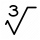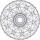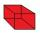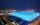# Cube + third power - math problems

#### Number of problems found: 70

• One third powerWhich equation justifies why ten to the one-third power equals the cube root of ten?
• Cube rootFind cube root of 18
• Cube edgesIf the edge length of the cube increases by 50%, how does the volume of this cube increase?
• Special cubeCalculate the edge of cube, if its surface and its volume is numerically equal number.
• Cube rootFor 13, Sam wrote 2891 instead of the correct cube number. By how much was he wrong?
• Cube 3How many times will increase the volume of a cube if we double the length of its edge?
• Cube in ballCube is inscribed into the sphere of radius 181 dm. How many percent is the volume of cube of the volume of the sphere?
• Cube V2SThe volume of the cube is 27 dm cubic. Calculate the surface of the cube.
• Cube 6Surface area of one wall cube is 1600 cm square. How many liters of water can fit into the cube?
• CubeThe cube weighs 11 kg. How weight is a cube of the same material if its dimensions are 3-times smaller?
• Cube basicsHow long is the edge length of a cube with volume 15 m3?
• The volume 2The volume of a cube is 27 cubic meters. Find the height of the cube.
• CubeThe sum of lengths of cube edges is 57 cm. What is its surface and volume?
• Cube and waterHow many liters of water can fit into a cube with an edge length of 0.11 m?
• CubeOne cube has edge increased 5 times. How many times will larger its surface area and volume?
• Big cubeCalculate the surface of the cube, which is composed of 64 small cubes with an edge 1 cm long.
• Cube-shaped boxThe cube-shaped box is filled to the brim with 2 liters of milk. Calculate the edge and surface of the box.
• Cube diagonalsCalculate the length of the side and the diagonals of the cube with a volume of 27 cm3.
• Cube surfce2volumeCalculate the volume of the cube if its surface is 150 cm2.
• LatheFrom the cube of edge, 37 cm was a lathed maximum cylinder. What percentage of the cube is left as waste after lathed?

Do you have an interesting mathematical word problem that you can't solve it? Submit a math problem, and we can try to solve it.

We will send a solution to your e-mail address. Solved examples are also published here. Please enter the e-mail correctly and check whether you don't have a full mailbox.

Please do not submit problems from current active competitions such as Mathematical Olympiad, correspondence seminars etc...

Cube Problems. Third power - math word problems.